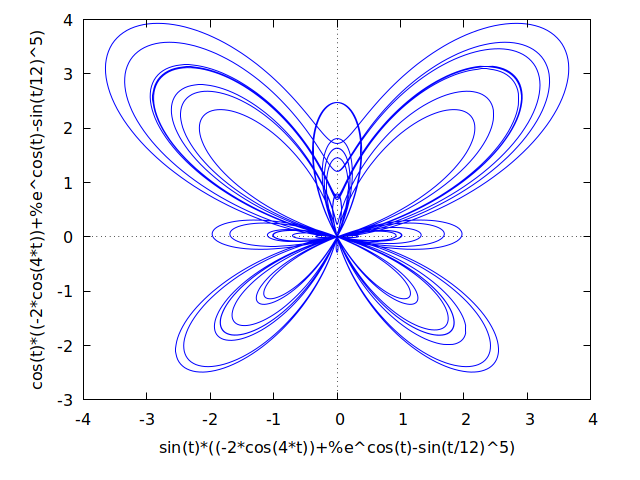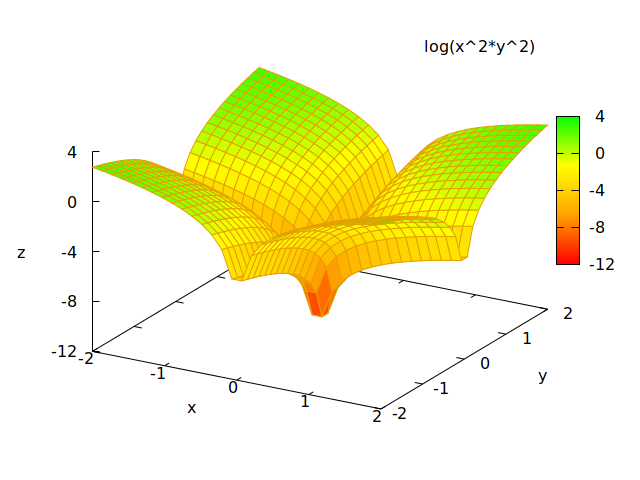Development version of the CRAN package. rim provides an interface to the powerful and fairly complete maxima computer algebra system

This repository is a fork from the original RMaxima created by Kseniia Shumelchyk and Hans W. Borcher shumelchyk/rmaxima, which is currently not maintained.

# Requirements

• Maxima (>= 5.42.1), this package has been tested with version between 5.42.1 and 5.46.0, but may also work with older versios

# Installation

To install the current release version from CRAN:

install.packages("rim")

If you want to install the latest version the easiest way is to install the R package remotes first and install the package from this github repo:

install.packages("remotes")
remotes::install_github(repo = "rcst/rim")

# Usage

If you want to learn how to use Maxima you can check out a number of ressources from it’s project site.

## RMarkdown

This section demonstrates the use of rim via it’s knitr engine. Alternatively, you can run each line of the Maxima code chunks via maxima.get().

This page has been generated using rim’s knitr engine itself.

Note that you can set the output format, e.g. maxima.options(engine.format = "latex") to get LaTeX-style expression rendering. When using pandoc to render the resulting file, MathJax is loaded or embedded automatically, when the ouput is a HTML document and so the Maxima output expressions are rendered nicely. Alternatively, expression outputs can be printed in MathML directly using maxima.options(engine.format = "mathml").

When attaching the package and Maxima is installed already, then it will be set up and started in the background automatically, when a command is send. In addition, if you have installed the R-package knitr (which get’s installed by default with this package as well), it will register maxima as a knitr engine. If this worked, maxima code can be used and rendered in RMarkdown documents.

library(rim)
maxima.options(engine.format = "latex",
engine.label = TRUE,
inline.format = "latex",
inline.label = FALSE)

For example, to generate this page you can download it’s source index.Rmd from here and then use rmarkdown::render to generate it.

download.file(url = "https://raw.githubusercontent.com/rcst/rim/master/docs/index.Rmd",
destfile = "index.Rmd")
rmarkdown::render("index.Rmd")

Now we can enter Maxima expression inside code chunks. Note that we need to end each line by ; or $ (suppressing output). For example we can type the following code chunk into our RMarkdown document file:  {maxima} L: sqrt(1 - 1/R^2); assume(R > 0)$
'integrate(x, x, 0, L) = integrate(x, x, 0, L);



In the above code chunk we define a variable L (that depends on another variable R). We tell Maximas database to assume R being larger than zero and suppress any output resulting from this command. The last line prints an unevaluated integral on the left-hand side of the equal sign and on the right-hand side evaluates that same definit integral. The tick quotation mark tells Maxima not to evaluate the suceeding expression. This, when rendered into a document will be printed as

(%i1) L: sqrt(1 - 1/R^2);

$\mathtt{(\textit{%o}_{1})}\quad \sqrt{1-\frac{1}{R^2}}$

(%i2) assume(R > 0)$(%i3) 'integrate(x, x, 0, L) = integrate(x, x, 0, L); $\mathtt{(\textit{%o}_{3})}\quad \int_{0}^{\sqrt{1-\frac{1}{R^2}}}{x\;dx}=\frac{R^2-1}{2\,R^2}$ The resulting output (if not suppressed) is printed after each line of code, and thus apprears as several. Input and output reference labels that are assign by Maxima are printed into he code chunk. Those can be used to refer to previous commands in other code chunks. For example (%i4) sqrt(rhs(%o3)); $\mathtt{(\textit{%o}_{4})}\quad \frac{\sqrt{R^2-1}}{\sqrt{2}\,R}$ takes the right-hand side of the result of the equation from input label %i3, which is assigned to output label %o3 and computes the square-root of it. Of course exercising on such simple computations is of little benefit. The real benefit comes from more complicated expression and the effort that we would need to put if we wanted to typeset the result, such as this (%i5) integrate(1 / (1 + x^4), x); $\mathtt{(\textit{%o}_{5})}\quad \frac{\log \left(x^2+\sqrt{2}\,x+1\right)}{2^{\frac{5}{2}}}-\frac{\log \left(x^2-\sqrt{2}\,x+1\right)}{2^{\frac{5}{2}}}+\frac{\arctan \left(\frac{2\,x+\sqrt{2}}{\sqrt{2}}\right)}{2^{\frac{3}{2}}}+\frac{\arctan \left(\frac{2\,x-\sqrt{2}}{\sqrt{2}}\right)}{2^{\frac{3}{2}}}$ pandoc automatically renders the LaTeX output format from Maxima by including MathJax JavaScript script. In general, pandoc takes care of how mathematical equations delimited by $$ are rendered. However, we can also change Maxima’s output format to MathML, which works if the output document is a HTML document. maxima.options(engine.format = "mathml") maxima.options() ##$format
##  "linear"
##
## $engine.format ##  "mathml" ## ##$inline.format
##  "inline"
##
## $label ##  TRUE ## ##$engine.label
##  TRUE
##
## $inline.label ##  FALSE (%i6) sqrt(3/4); (%i7) f(x) := e^(x^2)$
(%i8) diff(f(x), x);

(%i9) %;

Notice, that we can use the symbol % to refer to the last expression and that this works across code chunks.

You can also replay other previous expressions by referring to their output labels, as already demonstrated above. Whether or not reference labels are printed via the knitr engine has no influence on their existence in Maxima internally.

rim also supports printing Maxima output as inline. This works by putting an r inline code chunk and using maxima.inline(). Example: r maxima.inline("log(%o1);") results in $$\frac{\log \left(1-\frac{1}{R^2}\right)}{2}$$ .

maxima.options(engine.format = "latex")

### Chunk Options

The following chunk options are currently available:

• echo: Should the code chunk be printed (default is TRUE). If eval=FALSE, individual code lines are printed without without input labels, since these are assigned by Maxima upon evaluation
• eval: Should the code chunk be evaluated by Maxima (default is TRUE)
• include: whether code chunk and results should be printed (default is TRUE). If FALSE, code is still evaluated

NOTE: The results chunk option is explicitly ignored from chunk header and implicitly controlled via maxima.options(engine.format = ...).

# Demos

## Plots

(%i11) r: (exp(cos(t))-2*cos(4*t)-sin(t/12)^5)$(%i12) plot2d([parametric, r*sin(t), r*cos(t), [t,-8*%pi,8*%pi]]);plot2d() (%i13) plot3d(log (x^2*y^2), [x, -2, 2], [y, -2, 2],[grid, 29, 29], [palette, [gradient, red, orange, yellow, green]], color_bar, [xtics, 1], [ytics, 1], [ztics, 4], [color_bar_tics, 4]);plot3d() (%i14) example1: gr3d (title = "Controlling color range", enhanced3d = true, color = green, cbrange = [-3,10], explicit(x^2+y^2, x,-2,2,y,-2,2))$
(%i15) example2:
gr3d (title          = "Playing with tics in colorbox",
enhanced3d     = true,
color          = green,
cbtics         = {["High",10],["Medium",05],["Low",0]},
cbrange = [0, 10],
explicit(x^2+y^2, x,-2,2,y,-2,2))$(%i16) example3: gr3d (title = "Logarithmic scale to colors", enhanced3d = true, color = green, logcb = true, logz = true, palette = [-15,24,-9], explicit(exp(x^2-y^2), x,-2,2,y,-2,2))$
(%i17) draw(
dimensions = [500,1500],
example1, example2, example3);